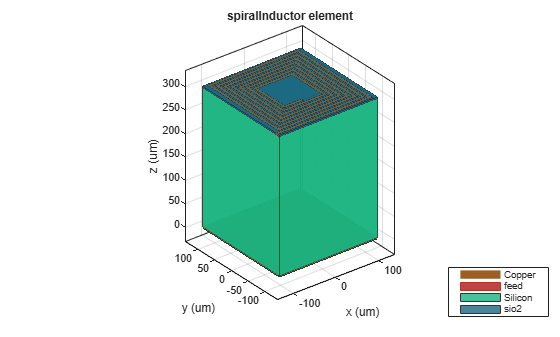# Characterization and Modelling of On-Chip Square Spiral Inductor for Si RFIC Application

This examples shows you how to model a square spiral inductor for Si (silicon) RFIC application.

The Silicon substrate is chosen for its low cost of Si IC fabrication over GaAs IC fabrication. The potential for integration with baseband circuits makes Si the process of choice in many RFIC applications.

The RF planar inductors play a vital role in wireless communication to meet the desired requirements like low supply voltage, low cost, low power dissipation, low noise, high frequency of operation, and low distortion.

The square spiral inductors are the most common in Si RF IC’s. Design the square spiral inductor over the Si substrate and the SiO2 insulator at 3 GHz.

#### Define Parameters

```N = 7; ID = 81e-6; W = 6e-6; S = 4e-6; % Height from the ground plane to the spiral H = 303e-6; GL = 230e-6; GW = 230e-6;```

#### Create Planer Square Spiral Inductor on Si and Sio2 Substrate

Model the 7 turn square spiral inductor is modelled using the defined parameters in microstrip line form on Silicon and Sio2 substrate

```Ind = spiralInductor; Ind.NumTurns = N; Ind.InnerDiameter = ID; Ind.Width = W; Ind.Spacing = S; Ind.Height = H; Ind.GroundPlaneLength = GL; Ind.GroundPlaneWidth = GW; d = dielectric('Name',{'Silicon','sio2'},'EpsilonR',[11.9,4.1],'LossTangent',[0.005,0],'Thickness',[300e-6,3e-6]); Ind.Substrate = d; figure; show(Ind);```Use the `sparameters` function to compute the s-parameters and plot it.

```spar = sparameters(Ind,linspace(1e9,5e9,21)); figure; rfplot(spar);```The s-parameters plot shows that after 430 MHz the S11 and S22 monotonically increases towards 0 dB. S12 and S21 decreases showing that energy is stored in inductor and not radiated. The behavior of S11 and S21 satisfies the law of conservation of energy.

Use the inductance function to calculate the measure of inductance of the square spiral inductor at 3 GHz.

`figure; inductance(Ind,linspace(1e9,5e9,21));`It is observed from the plot that the inductance of the spiral inductor increases with increase in frequency. Based on analyzing the Q- factor the peak in Q will be the design frequency of the inductor  , According to the reference the design frequency is around 3 GHz and the calculated inductance of the spiral is around 11.09 nH which is suitable for RFIC application.

```figure; mesh(Ind,'View','Metal');```Use the current function to plot the current distribution on the surface of the square spiral Inductor.

```figure; current(Ind,3e9,'scale','log10');```### Reference

1. Beeresha R Sa, A M Khanb, Manjunatha Reddy H Vc ,'' The Design and EM-Simulation of Square Spiral Inductor Using SimpleEquations'',Materials Today: Proceedings 5 (2018) 10875–10882.

2. Tuan Huu Bui, "Design and Optimization of a 10 nH Square-Spiral Inductor for Si RF Ics", University of North Carolina at Charlotte, October, 1999